# CHSE Odisha Class 11 Math Solutions Chapter 5 Principles Of Mathematical Induction Ex 5

Odisha State Board Elements of Mathematics Class 11 Solutions CHSE Odisha Chapter 5 Principles Of Mathematical Induction Ex 5 Textbook Exercise Questions and Answers.

## CHSE Odisha Class 11 Math Solutions Chapter 5 Principles Of Mathematical Induction Exercise 5

Prove the following by induction.

Question 1.
1 + 2 + 3 + …… + n = $$\frac{n(n+1)}{2}$$
Solution:
Let pn be the given statement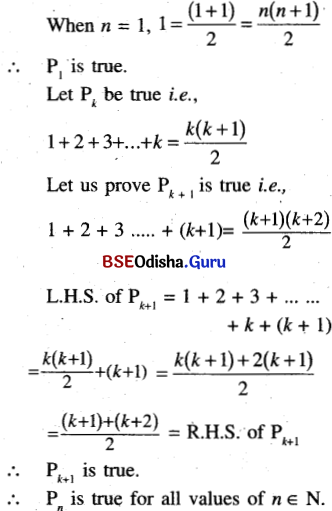Question 2.
12 + 22 + …… + n2 = $$\frac{n(n+1)(2 n+1)}{6}$$
Solution:
Let pn be the given statement
when n = 1
12 =1 = $$\frac{1(1+1)(2 \times 1+1)}{6}$$
P1 is true
Let Pk be true.
i.e. 12 + 22 + … + k2 = $$\frac{k(k+1)(2 k+1)}{6}$$
we shall prove Pk + 1 is true i.e., 12 + 22 + … + k2 + (k + 1)2
$$=\frac{(k+1)(k+2)(2 k+3)}{6}$$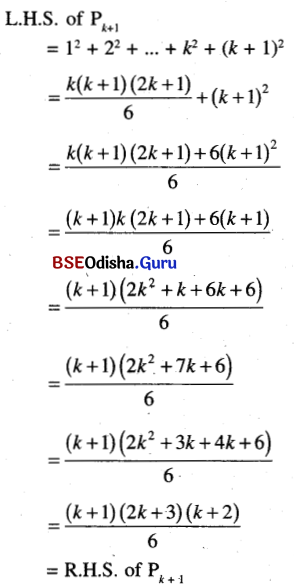∴ Pk+1 is true
∴ Pn is true of all values of n∈N.

Question 3.
1 + r + r2+ …. + rn = $$\frac{r^{n+1}-1}{r-1}$$
Solution:
Let Pn be the given statement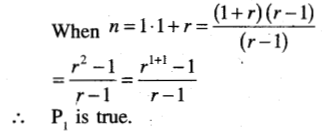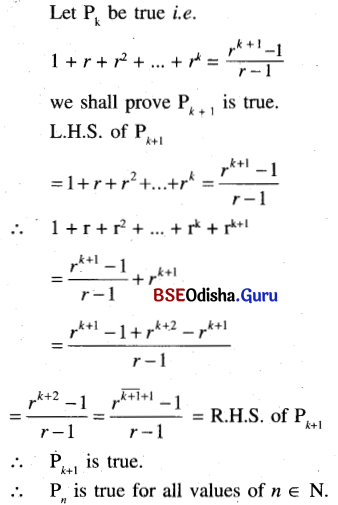Question 4.
5n – 1 is divisible by 4.
Solution:
Let Pn = 5n – 1
When n = 1,
51 – 1 = 4 is divisible by 4.
∴ P1, is true.
Let Pk be true i.e.,
5k – 1 is divisible by 4.
Let 5k+1 – 1 = 4m, me Z
Now 5k + 1 – 1 = 5k. 5 – 5 + 4
= 5 (5k – 1) + 4
= 5 × 4m + 4 = 4 (5m + 1)
which is divisible by 4.
∴ Pk+1 is true.
∴ Pn is true for all values of n ∈ NQuestion 5.
72n + 23n-3  3n-1 is divisible by 25 for any natural number n > 1.
Solution:
Let 72n + 23n-3 . 3n-1
when n = 1, 71 + 20 . 30 ⇒ 49 + 1 = 50
which is divisible by 25.
∴ P1 is true. Let Pk be true.
i.e., 72k + 23k-3 3k-1 is divisible by 35
Now $$7^{2 \overline{k+1}}+2^{3 \overline{k+1}-3} 3^{\overline{k+1}-1}$$
=72k+2 + 23k. 3k
= 72k. 72 + 23k-3 23. 3k-1. 31
= 72k. 49 + 23k-3. 3k-1. 24
= 72k (25 + 24) + 24. 23k-3. 3k-1
= 72k. 25 + 24 (72k + 23k-3. 3k-1)
= 72k. 25 + 24 × 25m
Which is divisible by 25 (∵ Pk is true)
∴ Pk+1 is true
∴ Pn is true for all values of n > 1.

Question 6.
7. 52n-1 + 23n+1 is divisible by 17 for every natural number n ≥ 1.
Solution:
Let Pn = 7. 52n – 1 + 23n+1.
When n = 1, 7.5 + 24 = 35 + 16 = 51
Which is divisible by 17.
P1, is true.
Let Pk be true i.e., 7.52k-1 + 23k+1  is divisible by 17.
Let 7.52k-1 + 23k+1 = 17 m, m ∈ Z
Now, $$7.5^{2 \overline{k+1}-1}+2^{3 \overline{k+1}+1}$$
= 7.52k-1 + 23k+4
= 7.52k-1 . 52 + 23k+1 . 23
= 25. 7. 52k-1 + 8. 23k+1
= (17 + 8) 7.52k-1 + 8. 23k+1
= 17. 7. 52k-1 + 8 (7. 52k-1 + 23k+1)
= 17 × 7 × 52k-1 + 8 × 17m
Which is divisible by 17.
Hence Pk+1. is true.
∴ Pn is true for all values of n ≥ 1.

Question 7.
4n+1 + 15n + 14 is divisible by 9 for every natural number n ≥ 0.
Solution:
Let Pn = 4n+1+ 15 n + 14
when n = 1, 42 + 15 + 14 = 45 is divisible by 9.
∴ P1 is true. Let Pk be true.
i.e., 4k+1 + 15k + 14 is divisible by 9.
Now, 4k+1+1 + 15 (k + 1) + 14
= 4k+2 + 15k + 29
= 4k+1. 4 + 60k + 56 – 45k – 27
= 4 (4k+1 + 15k + 14) – 9 (5k + 3)
Which is divisible by 9.
∴ Pk+1, is true.
∴ Pn is true for all values of n ≥ 0.

Question 8.
3(2n-1) + 7 is divisible by 9 for every natural number n ≥ 2.
Solution:
Let Pn = 32(n-1) + 7
When n = 2. 32 + 7 = 16 is divisible by 8.
∴ P2 is true.
Let Pk be true.
i.e., 52(k-1) + 7 is divisible by 8.
Let 32k-2 + 7 = 8m. m ∈ Z.
Now $$3^{2(\overline{k+1}-1)}$$ + 7 = 32k + 7
= 32k-2. 32 + 63 – 56
= 9(32k-2 + 7) – 56
= 9 × 8m – 56 = 8 (9m – 7)
Which is divisible by 8.
Pk+1 is true.
Pn is true for all values on n ≥ 2.

Question 9.
5(2n-4)  – 6n + 32 is divisible by 9 for every natural number n ≥ 5.
Solution:
Let P = 52(n-4) – 6n + 32
For n = 5, P5 = 52 – 6. 5 + 32
= 25 – 30 + 32 = 27
Which is divisible by 9.
Hence P5 is true.
Let Pk is true.
Let Pk is divisible by 9.
Let Pk = 52(k-4) – 6k + 32 = 9., m ≥ Z
52k+2 = 576 m + 24k  + 25 … (1)
we shall prove that Pk+1 is true.
Now 52(K+1)+2 – 24(k+1) – 25
= 52 (52k+2) – 24k – 24 – 25
= 52[576m + 24k + 25] – 24k – 24 – 25
= 25 × 576 m + 25 × 24k + 25 × 25 – 24k – 24 – 25
= 25 × 576 m + 576 k + 576
= 576 [25 m + k + 1]
which is divisible by 576
∴ Pk+1 is true.
So by the method of induction Pn is true for all n.
i.e., 52n+2  – 24n – 25 is divisible by 576 for all n ∈ N.
Hence Pk+1 is true.
So by methods of induction Pn is true.
i.e., 52n+2 – 24n – 25 is divisible by 576 for all n.Question 10.
$$\frac{1}{1.2}+\frac{1}{2.3}+\ldots+\frac{1}{n(n+1)}=\frac{n}{n+1}$$
Solution:
when n = 1,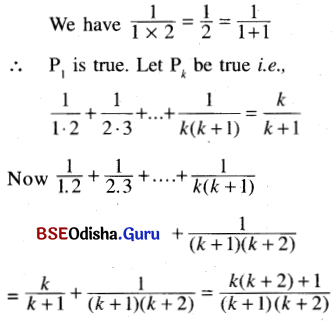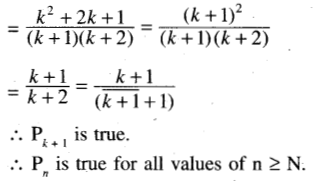Question 11.
1.3 + 2.4 + 3.5 + …….. + n(n + 2) = $$\frac{n(n+1)(2 n+7)}{6}$$
Solution:
when n = 1,
we have 1.3 = 3 = $$\frac{3 \times 6}{6}$$
$$=\frac{1 \times 2 \times 9}{6}=\frac{1(1+1)(2 \times 1+7)}{6}$$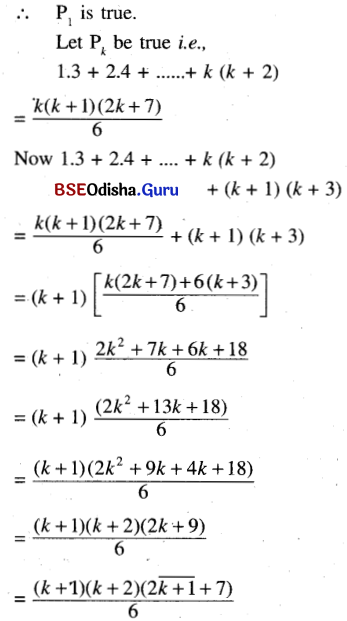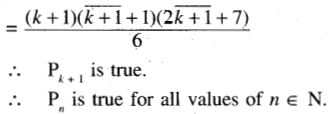Question 12.
xn – yn = (x – y)(xn-1 + xn-2 y + … + xyn-2 + yn-1); x, y ∈ R [Hint : Write xn+1 – yn+1 = x(xn – yn) + yn(x – y)]
Solution:
Let p(n) is
xn – yn = (x – y)(xn-1 + xn-2 y + … + xyn-2 + yn-1); x, y ∈ R
Step – 1:
For n = 2
x2 – y2 = (x – y) (x + y) (True)
∴ P(1) is true.
Step – 2:
Let P(k) is true.
i.e., xk – yk = (x – y)(xk-1 + xk-2y + … +xyk-2 + yk-1)
Step – 3:
Let us prove Pk+1 is true.
i.e., xk+1 – yk+1 = (x – y) (xk + xk-1y + … (xyk-1 + yk)
L.H.S. = xk+1 – yk+1
= xk+1 – xyk + xyk – yk+1
= x(xk – yk) + yk (x – y)
= x(x – y)(xk-1 + xk-2 y + … + xyk-2 + yk-1) + yk(x – y) [by (1)]
= (x – y) [xk + xk-1 y + … + xyk-2 + xyk-1 + yk]
= R.H.S.
∴ P(k+1) is true.
Step – 4:
By Principle Of Mathematical Induction P(n) is true for all n ∈ N.

Question 13.
1 + 3 + 5 + ……. +(2n – 1) = n2
Solution:
Let P(n) is : 1 + 3 + 5 + ……. +(2n – 1) = n2.
Step – 1:
For n = 2
L.H.S. = 1 + 3 = 4 = 22 (R.H.S)
∴ P(1) is true.
Step – 2:
Let P(k) is true.
i.e., 1 + 3 + 5 … + (2k – 1) = k2 …(1)
Step – 3:
We will prove that P(k + 1) is true
i.e., we want to prove.
1+ 3 + 5 + … + (2k – 1) + (2k + 1) = (k + 1)2
L.H.S. = 1 + 3 + 5 + … + (2k – 1) + (2k + 1)
= k2 + 2k + 1          [By – (1)]
= (k + 1)2 = R.H.S.
Step – 4:
By the Principle of Mathematical Induction P(n) is true for all n.
i.e., 1 + 3 + 5 ….+ (2n – 1) = n2Question 14.
n > n; n is a natural number.
Solution:
Let P(n) is 2n > n
Step – 1:
21 > 1 (True)
∴ P(1) is true.
Step – 2:
Let P(k) is true.
⇒ 2k > k
Step – 3:
We shall prove that P(k + 1) is true
i.e., 2k+1 > k + 1
Now 2k+1 = 2.2k > 2k ≥ k + 1 for k ∈ N.
∴ 2k+1 > k + 1
⇒ P(k + 1) is true.
Step – 4:
By the Principle of Mathematical Induction P(n) is true for all n.
i.e., 2n > n for n ∈ N

Question 15.
(1, 2, 3 … n)3  > 8 (13 + 23 + 33 + … + n3), for n > 3.
Solution:
Let P(n) is
(1, 2, 3 … n)3  > 8 (13 + 23 + 33 + … + n3), for n > 3.
Step – 1:
For n = 4
(1. 2. 3. 4)3 = 243 = 13824
8(13+ 23 + 33 + 43) = 808
∴ (1. 2 . 3 . 4)3 > 8(13 + 23 + 33 + 43)
∴ P(4) is true.
Step- 2:
Let P(k) is true.
(1. 2. 3…….k)3 > 8(13 + 23 + 33 + …… + k3)
Step – 3:
We shall prove that P(k+1) is true.
i.e., (1. 2. 3. …….. k(k+1))3 > 8(13 + 23 + … + k3 + (k + 1)3)
Now (1. 2. 3. …….. k(k + 1)3)
= (1. 2. 3 … k)3 (k + 1)3
> 8 (13 + 23 + … k3) (k + 1)3
> 8 (13 + 23 + … k3) + 8(k + 1)3
= 8 (13 + 23 + … + k3 + (k + 1)3)
P(k+1) is true.
Step – 4:
By the Principle of Mathematical Induction P(n) is true for all n ∈ N and n > 3.

Question 16.
$$\frac{1}{n+1}+\frac{1}{n+2}+\ldots+\frac{1}{3 n+1}>$$ for every positive integer n.
Solution:
Let P(n) is
$$\frac{1}{n+1}+\frac{1}{n+2}+\ldots+\frac{1}{3 n+1}>$$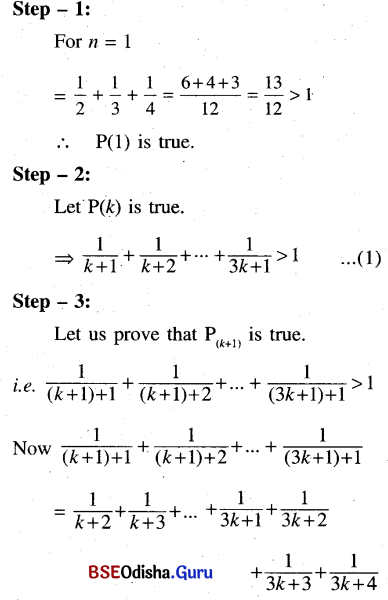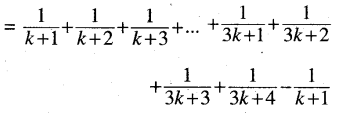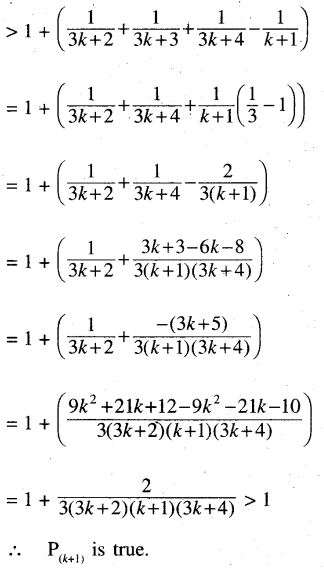Step-4:
By the Principle of Mathematical Induction P(n) is true for all n ∈ N.